# Difference between revisions of "QuickBird"

## Overview

Scenes are 16.5km x 16.5km.

Data is 11-bit integers stored in a 16-bit integer field. Thus band minimum = 0, band maximum = 2047.

Multi-spectral bands (2.44 - 2.88m pixel res)

band 1 = blue
2 = green
3 = red
4 = Near IR


Panchromatic band (61-72cm pixel res)

From the whitepaper Radiance Conversion of QuickBird Data:

QuickBird products are delivered to the customer as radiometrically corrected image pixels (qPixel,Band).

Radiometric correction includes a dark offset subtraction and a non-uniformity correction (detector-to-detector relative gain). Corrected counts are specific to the QuickBird instrument and therefore QuickBird imagery MUST be converted to spectral radiance before radiometric/spectral analysis or comparison with imagery from other sensors in

## Pre-Processing Overview

Followig needs some attention/review due to differences in terminology, i.e. Top-of-Atmrosphere Radiance as referenced in Radiometric Use of QuickBird Imagery, Technical Note, by Keith Krause (2005).

Typically, multispectral satellite data are converted into physical quantities such as Radiance or Reflectance before they are subjected in multispectral analysis techniques (image interpretation, band arithmetic, vegetation indices, matrix transformations, etc.). The latter can be differentiated in Top of Atmosphere Reflectance (ToAR) which does not account for atmospheric effects (absorption or scattering) and in Top of Canopy Reflectance (ToCR) which introduces a "correction" for atmospheric effects.

In order to derive Reflectance values, likewise as with remotely sensed data acquired by other sensors, IKONOS raw image digital numbers (DNs) need to be converted to at-sensor spectral Radiance values. At-sensor spectral Radiance values are an important input for the equation to derive Reflectance values. Note, Spectal Radiance is the measure of the quantity of radiation that hits the sensor and typically expressed in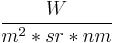$\frac{W}{m^2*sr*nm}$, that is watts per unit source area, per unit solid angle, and per unit wavelength.

The equation for the conversion to Top of Atmosphere Radiance, as provided in the document by Keith Krause (2005), Radiometric Use of QuickBird Imagery, Technical Note, is:

Failed to parse (lexing error): L\lambda = ( K_Band ⋅ q_(Pixel, Band) / ∆\lambda_Band )

 # where

 # LλPixel,Band:  Top-of-Atmosphere Spectral Radiance image pixels [W-m-2-sr-1-μm-1]
# KBand:  the absolute radiometric calibration factor [W-m-2-sr-1-count-1] for a given band
# qPixel,Band:  radiometrically corrected image pixels [counts  or  Digital Numbers]
# ∆λ_Band:  the effective bandwidth [μm] for a given band


• Converting QuickBird DNs to Radiance can be done by using the following equation: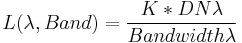$L(\lambda, Band) = \frac{K * DN\lambda}{Bandwidth\lambda}$
• Converting to Top of Atmosphere Reflectance, also referred to as Planetary Reflectance, can be done by using the following equation:$\rho_p = \frac{\pi * L\lambda * d^2}{ESUN\lambda * cos(\Theta_S)}$
where:
•$\rho$ - Unitless Planetary Reflectance
•$\pi$ - mathematical constant (3.14159265358)
•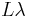$L\lambda$ spectral Radiance at the sensor's aperture, from equation... ToADD
•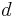$d$ - Earth-Sun distance in astronomical units, interpolated values
•$Esun$ - Mean solar exoatmospheric irradiance(s) (W/m2/μm), interpolated values
•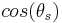$cos(\theta_s)$ - Solar_zenith_angle from the image acquisition's metadata

## Modules overview

• gdalinfo
• gdalwarp

### Inside GRASS GIS

• GeoTIFF, NITF 2.1, NITF 2.0
• Image bits / pixel: 8 or 16 bits

## Pre-Processing

### Importing data

Setting up a Location in GRASS GIS requires to know the Spatial Reference System in which the data of interest are projected. Retrieving the projection parameters of QuickBird imagery, can be done by using the gdalinfo program.

• Various ways of Location creation based on georeferenced data

Once inside an appropriate Location, importing the data is done with the r.in.gdal module.

For example, GeoTIFF files/bands, can be imported in one go by looping r.in.gdal over all of them

for TIF in echo *.tif; do r.in.gdal in=${TIF} out=${TIF%%.*}; done


### Cleanup

Rename the NIR band

g.rename QBird_Multichrom.4,QBird_Multichrom.NIR


Red and blue bands are swapped, so fix that by renaming them. (see )

g.rename QBird_Multichrom.blue,QBird_Multichrom.realred
g.rename QBird_Multichrom.red,QBird_Multichrom.blue
g.rename QBird_Multichrom.realred,QBird_Multichrom.red


No data is set to 0, so convert that to NULL with r.null:

for BAND in red green blue NIR ; do
r.null QBird_Multichrom.$BAND setnull=0 echo$BAND:
r.info -r QBird_Multichrom.$BAND echo done  Set color tables appropriately for 11-bit data: for BAND in red green blue NIR ; do r.colors QBird_Multichrom.$BAND color=rules << EOF
0 black
2047 white
EOF
done


It is not necessarily needed, but if you wish to rescale to 8-bit data you can use r.rescale as follows:

for BAND in red green blue NIR ; do
r.rescale in=QBird_Multichrom.$BAND from=0,2047 \ out=QBird_Multichrom255.$BAND to=0,255
done


Display the 3-band RGB image:

d.rgb r=QBird_Multichrom.red g=QBird_Multichrom.green b=QBird_Multichrom.blue


If the image is excessively dark you might try using the i.landsat.rgb module to auto-balance the colors and redraw:

i.landsat.rgb r=QBird_Multichrom.red g=QBird_Multichrom.green b=QBird_Multichrom.blue
d.redraw


### Deriving Physical Quantities

Converting Digital Numbers to Radiance/Reflectance requires knowledge about the sensor's specific spectral band parameters. Those are, as extracted from the document ...ToAdd:

Followig needs cleaning

# --------------------------------------------------------------------
# Table 5: Revised  K  Factors for   16-Bit   products
# Spectral Band (TDI Level) / K(revised) [W-m-2-sr-1-count-1]

# 1st column: k′ Conversion Factors for   16-Bit   products
# 2nd column: Effective Bandwidth [μm] per Spectral Band

Pan_10=0.08381880 # Pan_10=8.381880e-02
Pan_13=0.06447600	;	Pan_Width=0.398 # Pan_13=6.447600e-02
Pan_18=0.04656600 # Pan_18=4.656600e-02
Pan_24=0.03494440 # Pan_24=3.494440e-02
Pan_32=0.02618840 # Pan_32=2.618840e-02
K_Blue=0.01604120	;	Blue_Width=0.068 	# K_Blue=1.604120e-02
K_Green=0.01438470	;	Green_Width=0.099 	# K_Green=1.438470e-02
K_Red=0.01267350	;	Red_Width=0.071 	# K_Red=1.267350e-02
K_NIR=0.01542420	;	NIR_Width=0.114 	# K_NIR=1.542420e-02

# --------------------------------------------------------------------
# Table 6:  k′  Conversion Factors for   8-Bit   products
# Spectral Band / TDI Level / k′

# 1st column: k′ Conversion Factors for   8-Bit   products
# 2nd column: Effective Bandwidth [μm] per Spectral Band
Pan_10=1.02681367
Pan_13=1.02848939	;	Pan_Width=0.398
Pan_18=1.02794702
Pan_24=1.02989685
Pan_32=1.02739898
K_Blue=1.12097834	;	Blue_Width=0.068
K_Green=1.37652632	;	Green_Width=0.099
K_Red=1.30924587	;	Red_Width=0.071
K_NIR=0.98368622	;	NIR_Width=0.114


Converting a Blue Band (Digital Numbers) in to Spectral Radiance in the correct units to be further used for the conversion in to unitless Reflectance:

# set the region
g.region rast=Blue_DNs -p

# convert DNs to spectral Radiance values


#### Planetary Reflectance

The equation to derive Reflectance values incorporates in addition:

• The mathematical constant Pi PI=3.14159265358.
• The Earth-Sun distance in astronomical units which depends on the acquisition's day of year (DOY -- also referred to as Julian day, Ordinal_date) and can be retrieved from the following spreadsheet <http://landsathandbook.gsfc.nasa.gov/excel_docs/d.xls>.
• The mean solar exoatmospheric irradiance in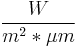$\frac{W}{m^2*\mu m}$. See 3rd column of (interplated) values given above.
• The cosine of the Solar Zenith Angle (SZA) at the time of the acquisition. The SZA can be calculated from its complementary Solar Elevation Angle (SEA) given in the image acquisition's metadata.

In the following example we accept as the acquisition's DOY=... and SEA=.... Hence, we get the Earth-Sun distance ESD=... and SZA = ... deg.

Converting the in-Blue spectral band at-sensor Radiance in to Planerary Reflectance:

PI=3.14159265358; ESD=...; BAND_Esun=...; SZA=...
r.mapcalc "Blue_Reflectance = ( ${PI} * Blue_Radiance *${ESD}^2 ) / ( ${BAND_Esun} * cos(${SZA}) )"


#### Automatising Conversions

The conversion process can be scripted to avoid repeating the same steps for each band separately.

## Post-Processing

Having beforehand satellite image data expressed in physical quantities (radiance or reflectance) is preferred to follow-up with digital image analysis techniques.

## Pan Sharpening

In GRASS ver. 6.x, one can use the i.fusion.brovey module.

i.fusion.brovey -q ms1=qbird_green ms2=qbird_nir ms3=qbird_red pan=qbird_pan outputprefix=brov